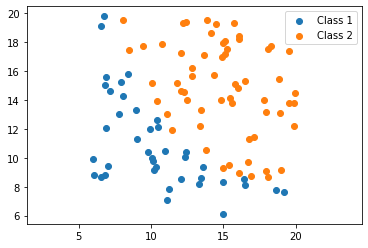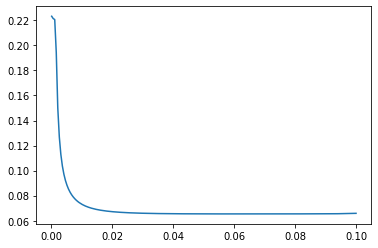# Lab 2: Logistic Regression¶

In this lab we will consider a student scores dataset.

First, import the mllab.py script.

In :
from mllab import *


## Question 1¶

The exams.txt file contains 3 columns that represent the exam 1, exam 2 scores and the result of 100 students (0: Not admitted, 1: Admitted).

• Open this file with a file editor to understand more the data.
• Load the data and check its size.
In :
students_results = np.loadtxt('exams.txt',delimiter='\t')

print(students_results.shape)

(100, 3)


## Question 2¶

• Extract exam 1 and exam 2 scores in a $(n,2)$-array and the labels (results) in a $(n,)$-array.
• Plot exam 1 against exam 2 with different color for each class of results.
• Hint: Look at the function $\verb!plotXY!$.
• Add a bias term and check data dimension.
In :
X = students_results[:,0:2]
y = students_results[:,2]

plotXY(X, y)
y = y.reshape(-1, 1)

print(X.shape, y.shape)

(100, 3) (100, 1)## Question 3¶

Implement the sigmoid function $$\sigma(z) = \left[ 1 + e^{-z} \right]^{-1}$$.

In :
def sigmoid(z):
return np.ones(z.shape)/(1+np.exp(-z))


## Question 4¶

Implement the MSE loss function associated and its gradient.

In :
def dMSE(y_true, y_pred):
return np.dot(X.T, y_pred-y_true)/X.shape

MSE_loss = (lambda beta : MSE(y, sigmoid(np.dot(X, beta))))
dMSE_loss = (lambda beta : dMSE(y, sigmoid(np.dot(X, beta))))


## Question 5¶

Find the best learning rate.

In :
Alpha = np.linspace(0.0001,0.1, 200)
Loss = list()
for alpha in Alpha:
beta_hat = grad_descent(dMSE_loss, *3, alpha=alpha, max_iter=1e5)
Loss.append(MSE_loss(beta_hat))
plt.plot(Alpha, Loss)
plt.show()## Question 6¶

Find the optimal weight parameter $\hat{\beta}$ with the gradient descent algorithm. Print the computation time.

In [ ]:



## Question 7¶

Plot the line separating the two classes.

In [ ]:



## Question 8¶

Implement the the log loss function: $$L(w)=\frac{1}{n}\sum_{i=1}^{n} \log \left[ 1 + e^{ -y_i (x_i \cdot \beta) } \right]$$ and its gradient.

In [ ]:



## Question 9¶

Find the best learning rate.

In [ ]:



## Question 10¶

Use gradient descent to find the optimal parameter. Print the computation time.

In [ ]:



## Question 11¶

Plot the line separating the two classes.

In [ ]:



## Question 12¶

Use the function $\verb!fmin_bfgs!$ to find $\hat{\beta}$. Print the computation time. Why is this a better solver ?

In [ ]:



## Question 13¶

Plot the line separating the two classes.

In [ ]:



## Question 14¶

Use $\verb!sklearn!$'s LogisticRegression. Plot the data as in Question 2. Plot the frontere line using the $\verb!plot_frontiere!$ function.

In [ ]:


In [ ]: